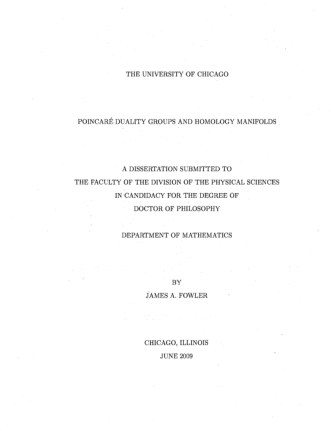# Ph.D. Thesis

A group $G$ is a Poincaré duality group if its classifying space $BG$ satisfies Poincaré duality; examples include fundamental groups of aspherical manifolds. C. T. C. Wall asked whether a Poincaré duality group is necessarily the fundamental group of an aspherical manifold, and M. Davis in [MR1747535] broadened Wall's question to $R$-homology manifolds:

Question.(M. Davis) Is every finitely presented torsion-free group satisfying Poincaré duality with $R$-coefficients (“$R$-PD”) the fundamental group of an aspherical closed $R$-homology manifold?

My thesis answers M. Davis' question in the negative, using Bestvina–Brady Morse theory to produce $\mathbb{Q}$-PD groups $G$ for which $BG$ does not have the homotopy type of a finite complex.

I also addressed a generalization version of M. Davis' question. There are weaker geometric conditions that nonetheless imply a group is $R$-PD: for $\pi$ to satisfy Poincaré duality with $R$ coefficients, it suffices that $\pi$ act freely on an $R$-acyclic $R$-homology manifold.

Question. Does every finitely presented group $\pi$ satisfying Poincaré duality with $R$ coefficients act freely on an $R$-acyclic $R$-homology manifold?

This is interesting when $R = \mathbb{Q}$. All finite groups are $0$-dimensional $\mathbb{Q}$-PD, and extensions of $\mathbb{Q}$-PD groups by finite groups are therefore $\mathbb{Q}$-PD. The restriction to torsion-free groups is not necessary, as groups with torsion may act freely on $\mathbb{Q}$-acyclic (albeit not contractible) finite complexes.

A particular class of $\mathbb{Q}$-PD groups to consider are uniform lattices with torsion (which, by Selberg, are extensions of manifold groups by finite groups).

Theorem.(Fowler) Let $\Gamma$ be a uniform lattice containing an element of finite order ($\neq$ 2). Although $\Gamma$ is $\mathbb{Q}$-PD, $\Gamma$ does not act freely on a $\mathbb{Q}$-acyclic $\mathbb{Q}$-homology manifold.

The proof involves two obstructions: a finiteness obstruction, and a controlled symmetric signature. With more work, I hope to address the situation for $\Gamma$ containing $2$-torsion

Sketch of Proof: The group $\Gamma$ is given as an extension $0 \to \pi \to \Gamma \to G \to 0$, where $\pi$ is torsion-free, and $G$ is a finite group. Given the Baum-Connes conjecture for $\Gamma$, the $\Gamma$-equivariant signature operator on $E\pi$ (which is the universal space for proper $\Gamma$ actions) cannot be lifted back to the signature operator on $B\Gamma$. To obstruct this lift, the space $E\pi$ is used to determine the equivariant signature operator of $\Gamma$ acting on the universal cover of the hypothetical homology manifold; geometric localization arguments can then be combined with calculations near fixed points to give a contradiction—although this last step fails in the absence of odd torsion.  ∎

In addition to controlled surgery considerations, there is a finiteness question:

Question. For a given group $G$, does there exist a free action of $G$ on an $R$-acyclic complex, with finite quotient?

Bestvina and Brady [MR1465330] call such groups FH over $R$, in analogy with other homological finiteness conditions.

In many cases, a finiteness obstruction will prevent $\Gamma$ from acting on a $\mathbb{Q}$-acyclic finite complex, let alone a $\mathbb{Q}$-homology manifold. Again, consider a uniform lattice $\Gamma$, and write $0 \to \pi \to \Gamma \to G \to 0$, with $\pi$ torsion-free and $G$ finite. For convenience, $G = \mathbb{Z}/p\mathbb{Z}$. If $G$ acts freely on a finite complex $B\pi$, then the Lefschetz fixed point theorem implies $\chi(B\pi^G) = 0$.

The equivariant finiteness obstruction fits into a categorical framework defined by Lück [MR1027600]; the condition $\chi(B\pi^G) = 0$, while necessary for $\Gamma$ to be FH, is not enough: $\chi(B\pi^G)$ can vanish while the Euler characteristics of the connected components of $B\pi^G$ may not vanish. If $\chi(C) = 0$ for each connected component $C$ of $B\pi^G$, then the equivariant finiteness obstruction does indeed vanish. The componentwise vanishing of the Euler characteristic is easy to verify in some cases, such as when the fixed sets are all odd-dimensional submanifolds.## References

Fowler, J. A. (2009). Poincare duality groups and homology manifolds (p. 60). ProQuest LLC, Ann Arbor, MI. Retrieved from http://gateway.proquest.com/openurl?url_ver=Z39.88-2004&rft_val_fmt=info:ofi/fmt:kev:mtx:dissertation&res_dat=xri:pqdiss&rft_dat=xri:pqdiss:3362018